Test: Probability & Statistics- 2

# Test: Probability & Statistics- 2 - Computer Science Engineering (CSE)

Test Description

## 30 Questions MCQ Test GATE Computer Science Engineering(CSE) 2024 Mock Test Series - Test: Probability & Statistics- 2

Test: Probability & Statistics- 2 for Computer Science Engineering (CSE) 2023 is part of GATE Computer Science Engineering(CSE) 2024 Mock Test Series preparation. The Test: Probability & Statistics- 2 questions and answers have been prepared according to the Computer Science Engineering (CSE) exam syllabus.The Test: Probability & Statistics- 2 MCQs are made for Computer Science Engineering (CSE) 2023 Exam. Find important definitions, questions, notes, meanings, examples, exercises, MCQs and online tests for Test: Probability & Statistics- 2 below.
Solutions of Test: Probability & Statistics- 2 questions in English are available as part of our GATE Computer Science Engineering(CSE) 2024 Mock Test Series for Computer Science Engineering (CSE) & Test: Probability & Statistics- 2 solutions in Hindi for GATE Computer Science Engineering(CSE) 2024 Mock Test Series course. Download more important topics, notes, lectures and mock test series for Computer Science Engineering (CSE) Exam by signing up for free. Attempt Test: Probability & Statistics- 2 | 30 questions in 90 minutes | Mock test for Computer Science Engineering (CSE) preparation | Free important questions MCQ to study GATE Computer Science Engineering(CSE) 2024 Mock Test Series for Computer Science Engineering (CSE) Exam | Download free PDF with solutions
 1 Crore+ students have signed up on EduRev. Have you?
Test: Probability & Statistics- 2 - Question 1

### A probability density function is of the form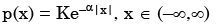The value of K is

Detailed Solution for Test: Probability & Statistics- 2 - Question 1

As (x) is a probability density function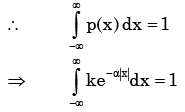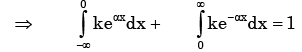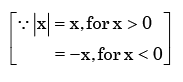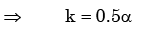Test: Probability & Statistics- 2 - Question 2

### If E denotes expectation, the variance of a random variable X is given by

Detailed Solution for Test: Probability & Statistics- 2 - Question 2

Varience of X = E{( X − m)2 }, m= mean of the distribution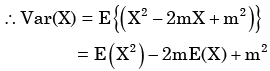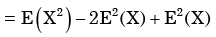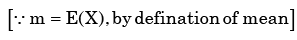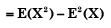Test: Probability & Statistics- 2 - Question 3

### Px(x) = M exp(–2|x|) – N exp(–3 |x|) is the probability density function for the real random variable X, over the entire x axis. M and N are both positive real numbers. The equation relating M and N is

Detailed Solution for Test: Probability & Statistics- 2 - Question 3

Given Px (x ) is the probability density function for the random variable X.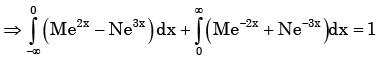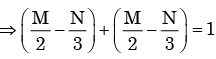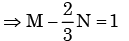Test: Probability & Statistics- 2 - Question 4

A fair coin is tossed independently four times. The probability of the event “the number of time heads shown up is more than the number of times tails shown up” is

Detailed Solution for Test: Probability & Statistics- 2 - Question 4

Here we have to find

P(H, H, H, T) + P(H, H, H, H)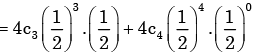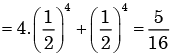Test: Probability & Statistics- 2 - Question 5

The standard deviation of a uniformly distributed random variable between 0 and 1 is

Detailed Solution for Test: Probability & Statistics- 2 - Question 5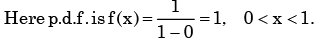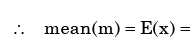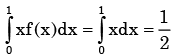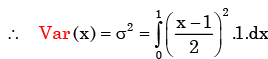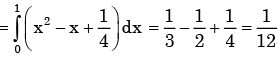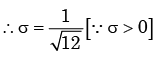Test: Probability & Statistics- 2 - Question 6

The probability of a defective piece being produced in a manufacturing process is 0.01. The probability that out of 5 successive pieces, only one is defective, is

Detailed Solution for Test: Probability & Statistics- 2 - Question 6

The required probability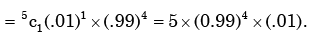Test: Probability & Statistics- 2 - Question 7

An unbiased coin is tossed three times. The probability that the head turns up in exactly two cases is

Detailed Solution for Test: Probability & Statistics- 2 - Question 7

Required probability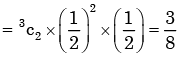Test: Probability & Statistics- 2 - Question 8

Manish has to travel from A to D changing buses at stops B and C enroute. The maximum waiting time at either stop can be 8 minutes each, but any time of waiting up to 8 minutes is equally likely at both places. He can afford up to 13 minutes of total waiting time if he is to arrive at D on time. What is the probability that Manish will arrive late at D?

Test: Probability & Statistics- 2 - Question 9

From a pack of regular from a playing cards, two cards are drawn at random. What is the probability that both cards will be Kings, if first card in NOT replaced

Detailed Solution for Test: Probability & Statistics- 2 - Question 9

Here sample space S = 52

∴The probability  of drawing both cards are king without replacement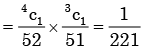Test: Probability & Statistics- 2 - Question 10

A single die is thrown twice. What is the probability that the sum is neither 8 nor 9?

Detailed Solution for Test: Probability & Statistics- 2 - Question 10

Here sample space = 36

Total No. of way in which sum is either 8 or 9  are

(2,6), (3,5),(3,6), (4,4), (4,5), (5,3), (5,4), (6,2), (6,3)

So  probability of getting sum 8 or 9 = 9/36

So the probability of not getting sum 8 or  9 = 1-1/4 = 3/4

Test: Probability & Statistics- 2 - Question 11

A coin is tossed 4 times. What is the probability of getting heads exactly 3 times?

Detailed Solution for Test: Probability & Statistics- 2 - Question 11

Probability of getting exactly three heads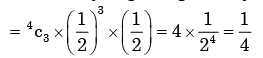Test: Probability & Statistics- 2 - Question 12

A box contains 2 washers, 3 nuts and 4 bolts. Items are drawn from the box at random one at a time without replacement. The probability of drawing 2 washers first followed by 3 nuts and subsequently the 4 bolts is

Detailed Solution for Test: Probability & Statistics- 2 - Question 12

Here sample space =  9

The required probability of drawing 2 washers, 3 nuts and 4 bolts respectively  without replac ement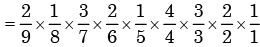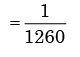Test: Probability & Statistics- 2 - Question 13

Analysis of variance is concerned with:

Detailed Solution for Test: Probability & Statistics- 2 - Question 13

Analysis of variance is used in comparing two or more populations, e.g. Different types of manures for yelding a single crop.

Test: Probability & Statistics- 2 - Question 14

A regression model is used to express a variable Y as a function of another variable X. This implies that

Test: Probability & Statistics- 2 - Question 15

A class of first year B. Tech. Students is composed of four batches A, B, C and D, each consisting of 30 students. It is found that the sessional marks of students in Engineering Drawing in batch C have a mean of 6.6 and standard deviation of 2.3. The mean and standard deviation of the marks for the entire class are 5.5 and 4.2, respectively. It is decided by the course instructor to normalize the marks of the students of all batches to have the same mean and standard deviation as that of the entire class. Due to this, the marks of a student in batch C are changed from 8.5 to

Detailed Solution for Test: Probability & Statistics- 2 - Question 15

Let mean and stander deviation of batch C be μc and σc respectively and mean  and standard deviation of entire class of 1st year students be μ and σ  respectively.

Given μ= 6.6 and σ = 2.3

and μ= 5.5 and σ = 4.2

In order to normalize batch C to entire class, the normalized score must be equated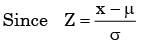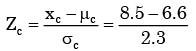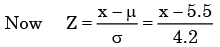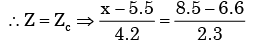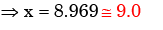Test: Probability & Statistics- 2 - Question 16

A hydraulic structure has four gates which operate independently. The probability of failure off each gate is 0.2. Given that gate 1 has failed, the probability that both gates 2 and 3 will fail is

Detailed Solution for Test: Probability & Statistics- 2 - Question 16

P(gate to and gate 3/gate 1 failed)

= P(gate2 and gate 3)

[ ∴ all three gates are independent corrosponding to each other]

=P(gate 2) ×P(gate 3)

=0.2 8*0.2 =0.04

Test: Probability & Statistics- 2 - Question 17

There are 25 calculators in a box. Two of them are defective. Suppose 5 calculators are           randomly picked for inspection (i.e., each has the same chance of being selected), what is the probability that only one of the defective calculators will be included in the inspection?

Detailed Solution for Test: Probability & Statistics- 2 - Question 17

Probability of only one is defective out of 5 calculators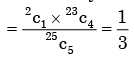Test: Probability & Statistics- 2 - Question 18

If probability density functions of a random variable X is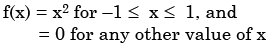Then, the percentage probability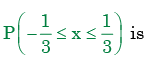Detailed Solution for Test: Probability & Statistics- 2 - Question 18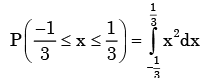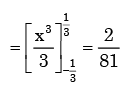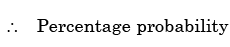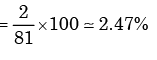Test: Probability & Statistics- 2 - Question 19

Two coins are simultaneously tossed. The probability of two heads simultaneously appearing is

Detailed Solution for Test: Probability & Statistics- 2 - Question 19

Probability of two head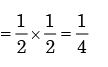Test: Probability & Statistics- 2 - Question 20

A fair coin is tossed three times in succession. If the first toss produces a head, then the probability of getting exactly two heads in three tosses is

Detailed Solution for Test: Probability & Statistics- 2 - Question 20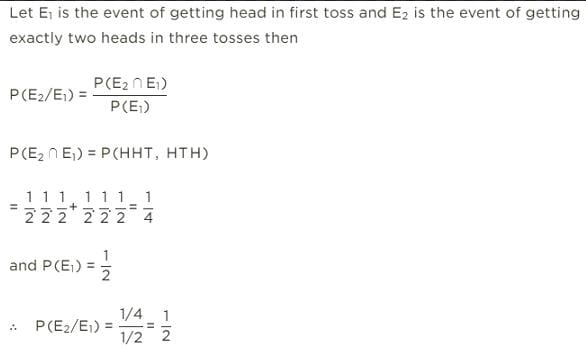Test: Probability & Statistics- 2 - Question 21

A box contains 4 white balls and 3 red balls. In succession, two balls are randomly selected and removed from the box. Given that the first removed ball is white, the probability that the second removed ball is red is

Detailed Solution for Test: Probability & Statistics- 2 - Question 21

After first ball is drawn white then sample space has 4 + 3 – 1 = 6 balls.  Probability of second ball is red without replacement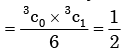Test: Probability & Statistics- 2 - Question 22

Consider a Gaussian distributed radom variable with zero mean and standard deviation σ. The value of its cumulative distribution function at the origin will be

Detailed Solution for Test: Probability & Statistics- 2 - Question 22

Gaussian distributed random variable is commonly known as normal distribution. The distribution is symmetrically distributed about mean and area under the curve is unity. Thus, for half the curve the area will be 0.5 (half of unity) since the curve is symmetric about zero here.

Test: Probability & Statistics- 2 - Question 23

The probability that there are 53 Sundays in a randomly chosen leap year is

Detailed Solution for Test: Probability & Statistics- 2 - Question 23

No. of days in a leap year are 366 days. In which there  are 52 complete weeks and 2 days  extra.

This 2 days may be of following combination.

1. Sunday & Monday

2. Monday & Tuesday

3. Tuesday & Wednesday

4. Wednesday & Thursday

5. Thursday & Friday

6. Friday & Saturday

7. Saturday & Sunday

There are two combination of Sunday in (1.) and (7).

∴ Re quired probability

=2/7

Test: Probability & Statistics- 2 - Question 24

Two dices are rolled simultaneously. The probability that the sum of digits on the top surface of the two dices is even, is

Detailed Solution for Test: Probability & Statistics- 2 - Question 24

Here sample space S= 6 × 6 = 36

Total no. of way in which sum of digits on the top surface of the two dice is is  even is  18.

∴ The require probability = 0.5

Test: Probability & Statistics- 2 - Question 25

The function y = sin φ, (φ > 0) is approximated as y = φ, where φ is in radian. The maximum value of φ for which the error due to the approximation is with in ±2% is

Detailed Solution for Test: Probability & Statistics- 2 - Question 25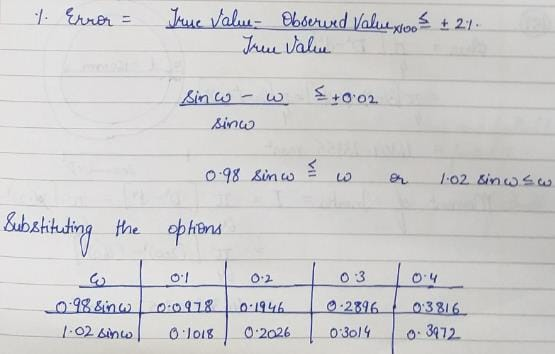Test: Probability & Statistics- 2 - Question 26

Aishwarya studies either computer science or mathematics everyday. if the studies computer science on a day, then the probability that she studies mathematics the next day is 0.6. If she studies mathematics on a day, then the probability that she studies computer science the next day is 0.4. Given that Aishwarya studies computer science on Monday, what is the probability that she studies computer science on Wednesday?

Detailed Solution for Test: Probability & Statistics- 2 - Question 26

Let C denote computes science study and M denotes maths study.

P(C on monday and C on wednesday)

= p(C on monday, M on tuesday and C on wednesday)

+ p(C on monday, C on tuesday and C on wednesday)

= 1 × 0.6 × 0.4 + 1 × 0.4 × 0.4

= 0.24 + 0.16

= 0.40

Test: Probability & Statistics- 2 - Question 27

An examination paper has 150 multiple-choice questions of one mark each, with each question having four choices. Each incorrect answer fetches – 0.25 mark. Suppose 1000 students choose all their answers randomly with uniform probability. The sum total of the expected marks obtained all these students is

Detailed Solution for Test: Probability & Statistics- 2 - Question 27

Let the marks obtained per question be a random variable X. It’s probability  distribution table is given below: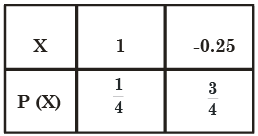Expected mark per question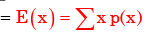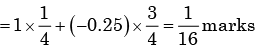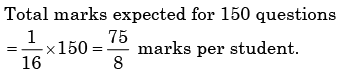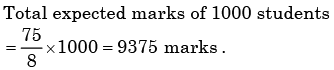Test: Probability & Statistics- 2 - Question 28

Let f(x) be the continuous probability density function of a random variable X. The probability that a < X ≤ b, is

Detailed Solution for Test: Probability & Statistics- 2 - Question 28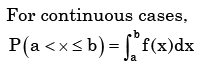Test: Probability & Statistics- 2 - Question 29

Let P(E) denote the probability of the even E. Given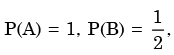the values of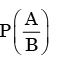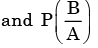respectively are

Detailed Solution for Test: Probability & Statistics- 2 - Question 29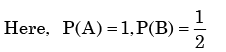We need to find the conditional probability of two given events without being told about P(AB). Also it is not mentioned that they are independent events. But since P(A)=1, it means that A covers the complete sample.

So, P(AB)=P(B)=1/2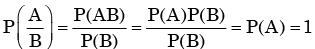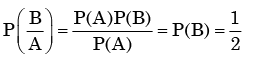Test: Probability & Statistics- 2 - Question 30

For each element is a set of size 2n, an unbiased coin is tossed. The 2n coin tossed are independent. An element is chosen if the corresponding coin toss were head. The probability that exactly n elements are chosen is

Detailed Solution for Test: Probability & Statistics- 2 - Question 30

The probability that exactly n elements are chosen

= the probability of getting n heads out of 2n tosses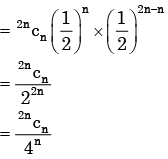## GATE Computer Science Engineering(CSE) 2024 Mock Test Series

151 docs|216 tests
Information about Test: Probability & Statistics- 2 Page
In this test you can find the Exam questions for Test: Probability & Statistics- 2 solved & explained in the simplest way possible. Besides giving Questions and answers for Test: Probability & Statistics- 2, EduRev gives you an ample number of Online tests for practice

## GATE Computer Science Engineering(CSE) 2024 Mock Test Series

151 docs|216 tests